# Fraction calculator

The calculator performs basic and advanced operations with fractions, expressions with fractions combined with integers, decimals, and mixed numbers. Also shows detailed step-by-step information about fraction calculation procedure. Solve problems with two, three or more fractions and numbers in one expression.

## Result:

### 5/100 = 1/20 = 0.05

Spelled result in words is one twentieth.

#### Rules for expressions with fractions:

Fractions - use the slash “/” between the numerator and denominator, i.e. for five-hundredths enter 5/100. If you are using mixed numbers be sure to leave a single space between the whole number and fraction part.
The slash separates the numerator (number above a fraction line) and denominator (number below).

Mixed numerals (mixed fractions or mixed numbers) write as non-zero integer separated by one space and fraction i.e., 1 2/3 (having the same sign). An example of a negative mixed fraction: -5 1/2.
Because slash is both signs for fraction line and division, we recommended use colon (:) as operator of division fractions i.e., 1/2 : 3.

Decimals (decimal numbers) enter with a decimal point . and they are automatically converted to fractions - i.e. 1.45.

Colon : and slash / is the symbol of division. Can be used to divide mixed numbers 1 2/3 : 4 3/8 or can be used for write complex fractions i.e. 1/2 : 1/3.
An asterisk * or × is the symbol for multiplication.
Plus + is addition, minus sign - is subtraction and ()[] is mathematical parentheses.
The exponentiation/power symbol is ^ - for example: (7/8-4/5)^2 = (7/8-4/5)2

#### Examples:

subtracting fractions: 2/3 - 1/2
multiplying fractions: 7/8 * 3/9
dividing Fractions: 1/2 : 3/4
exponentiation of fraction: 3/5^3
fractional exponents: 16 ^ 1/2
adding fractions and mixed numbers: 8/5 + 6 2/7
dividing integer and fraction: 5 ÷ 1/2
complex fractions: 5/8 : 2 2/3
decimal to fraction: 0.625
Fraction to Decimal: 1/4
Fraction to Percent: 1/8 %
comparing fractions: 1/4 2/3
multiplying a fraction by a whole number: 6 * 3/4
square root of a fraction: sqrt(1/16)
reducing or simplifying the fraction (simplification) - dividing the numerator and denominator of a fraction by the same non-zero number - equivalent fraction: 4/22
expression with brackets: 1/3 * (1/2 - 3 3/8)
compound fraction: 3/4 of 5/7
fractions multiple: 2/3 of 3/5
divide to find the quotient: 3/5 ÷ 2/3

Calculator follows well-known rules for order of operations. Most common mnemonics for remembering this order of operations are:
PEMDAS - Parentheses, Exponents, Multiplication, Division, Addition, Subtraction.
BEDMAS - Brackets, Exponents, Division, Multiplication, Addition, Subtraction
BODMAS - Brackets, Of or Order, Division, Multiplication, Addition, Subtraction.
GEMDAS - Grouping Symbols - brackets (){}, Exponents, Multiplication, Division, Addition, Subtraction.
Be careful, always do multiplication and division before addition and subtraction. Some operators (+ and -) and (* and /) has the same priority and then must evaluate from left to right.

## Fractions in word problems:

• A baker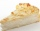A baker has 5 1/4 pies in her shop. She cut the pies in pieces that are each 1/8 of a whole pie. How many pieces of pie does she have?2 and 1 8th plus 1 and 1 3rd =
• Unknown number 23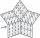Find 2/3 of unknown number, which is two thirds of the 99.Mom bake cookies. Rolo took 2/9 of all cookies, Michal 3/9. How many cookies ate Rolo if Michal had 9.
• Bricklayers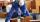8 bricklayers build a house for 630 days. How many bricklayers have to add after 150 days, so then the whole building completed in (next) 320 days?
• Tailor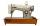Tailor bought 2 3/4 meters of textile and paid 638 CZK. Determine the price per 1 m of the textile.
• Cakes1/3 poppy cake, 1/3 apple, 15 pieces of cheese. How many are totally cakes?
• Pupil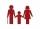I'm a primary school pupil. I attended the exercises of parents with children 1/4 of my age, 1/3 for drawing, and 1/6 for flute. For the first three years of my life, I had no ring, and I never went to two rings at the same time. How old am I?
• Roses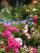On the large rosary was a third white, half red, yellow quarter and six pink. How many roses was in the rosary?
• Doug bikedDoug biked 5 1/4 miles in 3/4 of an hour. What is his average speed?
• Gold inheritance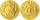The king divided the inheritance with his three sons in the ratio of 7: 6: 4. Two of them received 286,000 gold. How much each of the sons got.
• Birthday party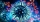For her youngest son's birthday party, mother bought 6 3/4 kg of hotdog and 5 1/3 dozens bread rolls. Hotdogs cost 160 per kilogram and a dozen bread roll costs 25. How much did she spend in all?
• Potatoes 6The farmer brought potatoes to the market. In the first hour he sold two-fifths of the potatoes brought, in the second hour he sold five-sixth of the remaining potatoes, and in the third hour he had sold the last 40kg of potatoes. How many kg of potatoes